1595414580

# Music Genre Classification using Transfer Learning(Pytorch)

My work is an extension of Pankaj Kumar’s work that can be found here. Instead of a feed-forward Neural Net, I used a pre-trained ResNet model(Transfer Learning) to gain better accuracy. (Thanks a lot Pankaj Kumar).

You can download the dataset from here. The dataset consists of 1000 audio tracks each 30 seconds long. It contains 10 genres, each represented by 100 tracks. The tracks are all 22050 Hz monophonic 16-bit audio files in .wav format.

The dataset consists of 10 genres i.e

• Blues
• Classical
• Country
• Disco
• Hip-hop
• Jazz
• Metal
• Pop
• Reggae
• Rock

# Let’s start

``````!mkdir genres && wget http://opihi.cs.uvic.ca/sound/genres.tar.gz && tar -xf genres.tar.gz genres/
``````

This helps to download the data and unpack it into a folder called ‘genres’.

Now, Let’s import the libraries we will be needing:

``````import torch
import torchvision
import torchaudio
import random
import numpy as np
import librosa
import librosa.display
import pandas as pd
import os
from PIL import Image
import pathlib
import matplotlib.pyplot as plt
import torch.nn as nn
import torch.nn.functional as F
from torchvision.transforms import ToTensor
from torchvision.utils import make_grid
from torch.utils.data import random_split
%matplotlib inline
from tqdm.autonotebook import tqdm
import IPython.display as ipd
import torchvision.transforms as T
``````

Now, I will give the data directory:

``````data_path = '/content/genres/'
``````

Now we will convert the music files i.e .wav files to image format using the Librosa library. A detailed explanation can be found here.

``````cmap = plt.get_cmap('inferno')

plt.figure(figsize=(8,8))
genres = 'blues classical country disco hiphop jazz metal pop reggae rock'.split()
for g in genres:
pathlib.Path(f'img_data/{g}').mkdir(parents=True, exist_ok=True)
for filename in os.listdir(f'{data_path}/{g}'):
songname = f'{data_path}/{g}/{filename}'
y, sr = librosa.load(songname, mono=True, duration=5)
plt.specgram(y, NFFT=2048, Fs=2, Fc=0, noverlap=128, cmap=cmap, sides='default', mode='default', scale='dB');
plt.axis('off');
plt.savefig(f'img_data/{g}/{filename[:-3].replace(".", "")}.png')
plt.clf()
``````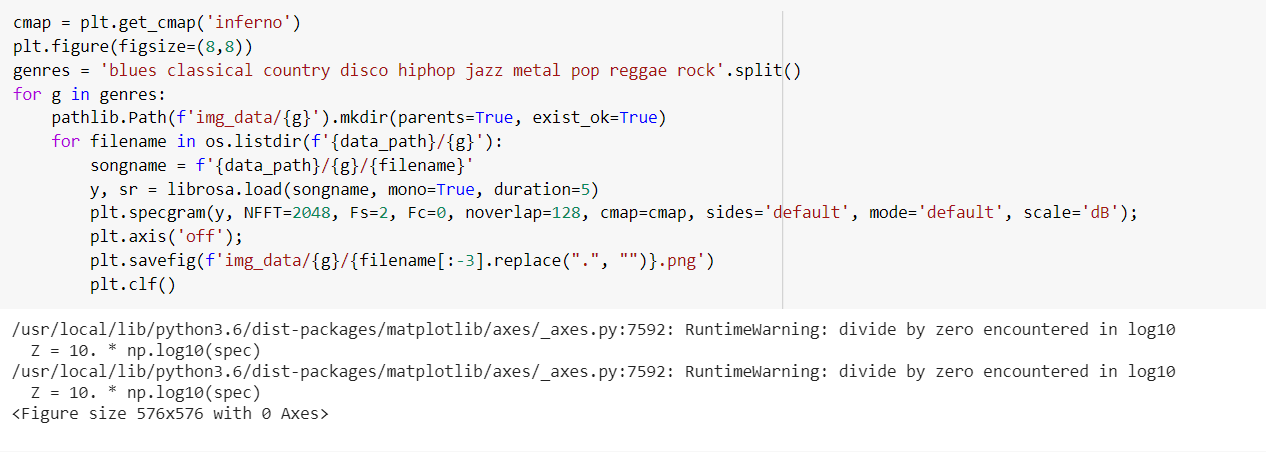Code Screenshot

Let’s visualize an image:

``````import matplotlib.image as mpimg
imgplot = plt.imshow(img)
plt.show()
print('shape of image is:',img.shape)
``````

#zero-to-gan #music-genre #deep-learning #pytorch #deep learning

## Buddha Community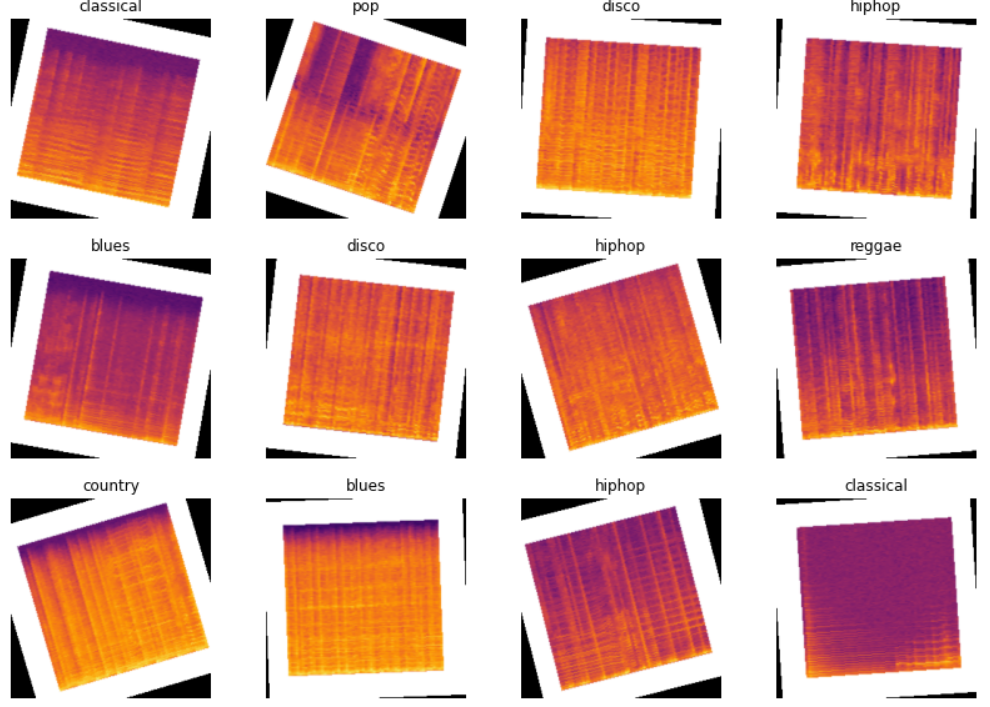1598891580

## Google Reveals "What is being Transferred” in Transfer Learning

Recently, researchers from Google proposed the solution of a very fundamental question in the machine learning community — What is being transferred in Transfer Learning? They explained various tools and analyses to address the fundamental question.

The ability to transfer the domain knowledge of one machine in which it is trained on to another where the data is usually scarce is one of the desired capabilities for machines. Researchers around the globe have been using transfer learning in various deep learning applications, including object detection, image classification, medical imaging tasks, among others.

#developers corner #learn transfer learning #machine learning #transfer learning #transfer learning methods #transfer learning resources1595414580

## Music Genre Classification using Transfer Learning(Pytorch)

My work is an extension of Pankaj Kumar’s work that can be found here. Instead of a feed-forward Neural Net, I used a pre-trained ResNet model(Transfer Learning) to gain better accuracy. (Thanks a lot Pankaj Kumar).

You can download the dataset from here. The dataset consists of 1000 audio tracks each 30 seconds long. It contains 10 genres, each represented by 100 tracks. The tracks are all 22050 Hz monophonic 16-bit audio files in .wav format.

The dataset consists of 10 genres i.e

• Blues
• Classical
• Country
• Disco
• Hip-hop
• Jazz
• Metal
• Pop
• Reggae
• Rock

# Let’s start

``````!mkdir genres && wget http://opihi.cs.uvic.ca/sound/genres.tar.gz && tar -xf genres.tar.gz genres/
``````

This helps to download the data and unpack it into a folder called ‘genres’.

Now, Let’s import the libraries we will be needing:

``````import torch
import torchvision
import torchaudio
import random
import numpy as np
import librosa
import librosa.display
import pandas as pd
import os
from PIL import Image
import pathlib
import matplotlib.pyplot as plt
import torch.nn as nn
import torch.nn.functional as F
from torchvision.transforms import ToTensor
from torchvision.utils import make_grid
from torch.utils.data import random_split
%matplotlib inline
from tqdm.autonotebook import tqdm
import IPython.display as ipd
import torchvision.transforms as T
``````

Now, I will give the data directory:

``````data_path = '/content/genres/'
``````

Now we will convert the music files i.e .wav files to image format using the Librosa library. A detailed explanation can be found here.

``````cmap = plt.get_cmap('inferno')

plt.figure(figsize=(8,8))
genres = 'blues classical country disco hiphop jazz metal pop reggae rock'.split()
for g in genres:
pathlib.Path(f'img_data/{g}').mkdir(parents=True, exist_ok=True)
for filename in os.listdir(f'{data_path}/{g}'):
songname = f'{data_path}/{g}/{filename}'
y, sr = librosa.load(songname, mono=True, duration=5)
plt.specgram(y, NFFT=2048, Fs=2, Fc=0, noverlap=128, cmap=cmap, sides='default', mode='default', scale='dB');
plt.axis('off');
plt.savefig(f'img_data/{g}/{filename[:-3].replace(".", "")}.png')
plt.clf()
``````Code Screenshot

Let’s visualize an image:

``````import matplotlib.image as mpimg
imgplot = plt.imshow(img)
plt.show()
print('shape of image is:',img.shape)
``````

#zero-to-gan #music-genre #deep-learning #pytorch #deep learning1596615120

## Transfer Learning Using ULMFit

This is the third part of a series of posts showing the improvements in NLP modeling approaches. We have seen the use of traditional techniques like Bag of Words, TF-IDF, then moved on to RNNs and LSTMs. This time we’ll look into one of the pivotal shifts in approaching NLP Tasks — Transfer Learning!

The complete code for this tutorial is available at this Kaggle Kernel

# ULMFit

The idea of using Transfer Learning is quite new in NLP Tasks, while it has been quite prominently used in Computer Vision tasks! This new way of looking at NLP was first proposed by Howard Jeremy, and has transformed the way we looked at data previously!

The core idea is two-fold — using generative pre-trained Language Model + task-specific fine-tuning was first explored in ULMFiT (Howard & Ruder, 2018), directly motivated by the success of using ImageNet pre-training for computer vision tasks. The base model is AWD-LSTM.

A Language Model is exactly like it sounds — the output of this model is to predict the next word of a sentence. The goal is to have a model that can understand the semantics, grammar, and unique structure of a language.

ULMFit follows three steps to achieve good transfer learning results on downstream language classification tasks:

1. General Language Model pre-training: on Wikipedia text.
2. Target task Language Model fine-tuning: ULMFiT proposed two training techniques for stabilizing the fine-tuning process.
3. Target task classifier fine-tuning: The pretrained LM is augmented with two standard feed-forward layers and a softmax normalization at the end to predict a target label distribution.

# Using fast.ai for NLP -

fast.ai’s motto — Making Neural Networks Uncool again — tells you a lot about their approach ;) Implementation of these models is remarkably simple and intuitive, and with good documentation, you can easily find a solution if you get stuck anywhere. Along with this, and a few other reasons I elaborate below, I decided to try out the fast.ai library which is built on top of PyTorch instead of Keras. Despite being used to working in Keras, I didn’t find it difficult to navigate fast.ai and the learning curve is quite fast to implement advanced things as well!

In addition to its simplicity, there are some advantages of using fast.ai’s implementation -

• Discriminative fine-tuning is motivated by the fact that different layers of LM capture different types of information (see discussion above). ULMFiT proposed to tune each layer with different learning rates, {η1,…,ηℓ,…,ηL}, where η is the base learning rate for the first layer, ηℓ is for the ℓ-th layer and there are L layers in total.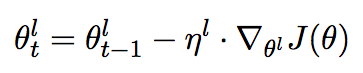Weight update for Stochastic Gradient Descent (SGD). ∇θ(ℓ)J(θ) is the gradient of Loss Function with respect to θ(ℓ). η(ℓ) is the learning rate of the ℓ-th layer.

• Slanted triangular learning rates (STLR) refer to a special learning rate scheduling that first linearly increases the learning rate and then linearly decays it. The increase stage is short so that the model can converge to a parameter space suitable for the task fast, while the decay period is long allowing for better fine-tuning.

#nlp #machine-learning #transfer-learning #deep-learning #sentiment-classification #deep learning1593702540

## Transfer Learning in Image Classification

The term Transfer Learning refers to the leverage of knowledge gained by a Neural Network trained on a certain (usually large) available dataset for solving new tasks for which few training examples are available, integrating the existing knowledge with the new one learned from the few examples of the task-specific dataset. Transfer Learning is thus commonly used, often together with other techniques such as Data Augmentation, in order to address the problem of lack of training data.

But, in practice, how much can Transfer Learning actually help, and how many training examples do we really need in order for it to be effective?

In this story, I try to answer these questions by applying two Transfer Learning techniques (e.g. Feature Extraction and Fine-Tuning) for addressing an Image Classification task, varying the number of examples on which the models are trained in order to see how the lack of data affects the effectiveness of the adopted approaches.

# Experimental Case Study

The task chosen for experimenting Transfer Learning consists of the classification of flower images into 102 different categories. The choice of this task is mainly due to the easy availability of a flowers dataset, as well as to the domain of the problem, which is generic enough to be suitable for effectively applying Transfer Learning with neural networks pre-trained on the well-known ImageNet dataset.

The adopted dataset is the 102 Category Flower Dataset created by M. Nilsback and A. Zisserman , which is a collection of 8189 labelled flowers images belonging to 102 different classes. For each class, there are between 40 and 258 instances and all the dataset images have significant scale, pose and light variations. The detailed list of the 102 categories together with the respective number of instances is available here.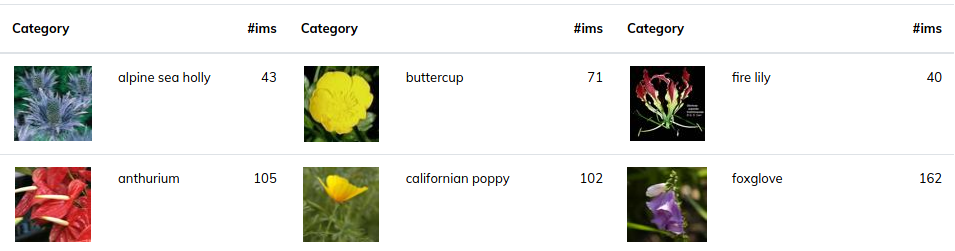Figure 1: Examples of images extracted from the 102 Category Dataset.

In order to create training datasets of different sizes and evaluate how they affect the performance of the trained networks, the original set of flowers images is split into training, validation and test sets several times, each time adopting different split percentages. Specifically, three different training sets are created (that from now on will be referred to as the LargeMedium and Small training sets) using the percentages shown in the table below.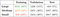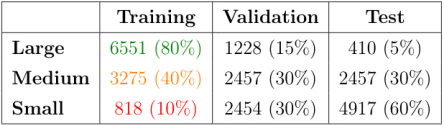Table 1: number of examples and split percentages (referred to the complete unpartitioned flowers dataset) of the datasets used to perform the experiments.

All the splits are performed adopting stratified sampling, in order to avoid introducing sampling biases and ensuring in this way that all the obtained training, validation and test subsets are representative of the whole initial set of images.

The image classification task described above is addressed by adopting the two popular techniques that are commonly used when applying Transfer Learning with pre-trained CNNs, namely Feature Extraction and Fine-Tuning.

## Feature Extraction

Feature Extraction basically consists of taking the convolutional base of a previously trained network, running the target data through it and training a new classifier on top of the output, as summarized in the figure below.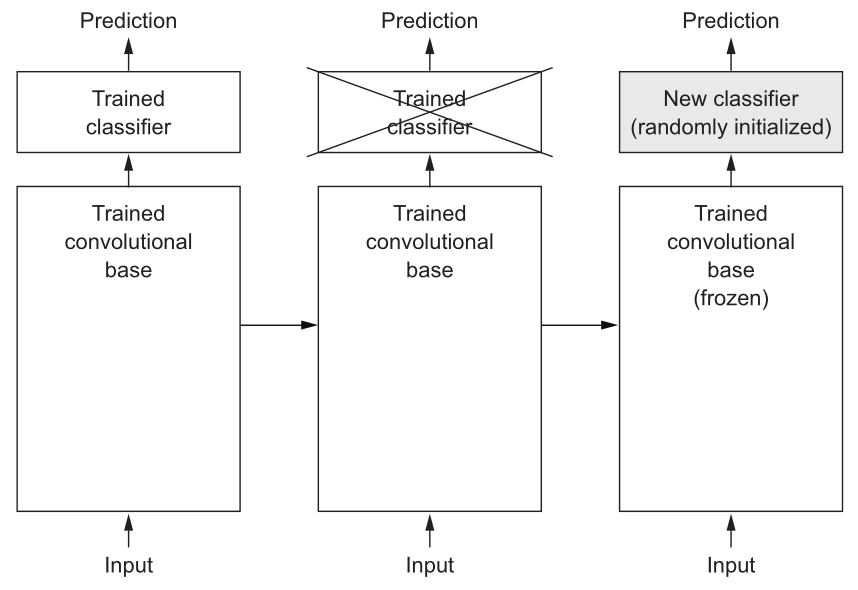Figure 2: Feature Extraction applied to a convolutional neural network: the classifiers are swapped while the same convolutional base is kept. “Frozen” means that the weighs are not updated during training.

The classifier stacked on top of the convolutional base can either be a stack of fully-connected layers or just a single Global Pooling layer, both followed by Dense layer with softmax activation function. There is no specific rule regarding which kind of classifier should be adopted, but, as described by Lin et. al , using just a single Global Pooling layer generally leads to less overfitting since in this layer there are no parameters to optimize.

Consequently, since the training sets used in the experiments are relatively small, the chosen classifier only consists of a single Global Average Pooling layer which output is fed directly into a softmax activated layer that outputs the probabilities for each of the 102 flowers categories.

During the training, only the weights of the top classifiers are updated, while the weights of the convolutional base are “frozen” and thus kept unchanged.

In this way, the shallow classifier learns how to classify the flower images into the possible 102 categories from the off-the-shelf representations previously learned by the source model for its domain. If the source and the target domains are similar, then these representations are likely to be useful to the classifier and the transferred knowledge can thus bring an improvement to its performance once it is trained.

## Fine-Tuning

Fine-Tuning can be seen as a further step than Feature Extraction that consists of selectively retraining some of the top layers of the convolutional base previously used for extracting features. In this way, the more abstract representations of the source model learned by its last layers are slightly adjusted to make them more relevant for the target problem.

This can be achieved by unfreezing some of the top layers of the convolutional base, keeping frozen all its other layers and jointly training the convolutional base with the same classifier previously used for Feature Extraction, as represented in the figure below.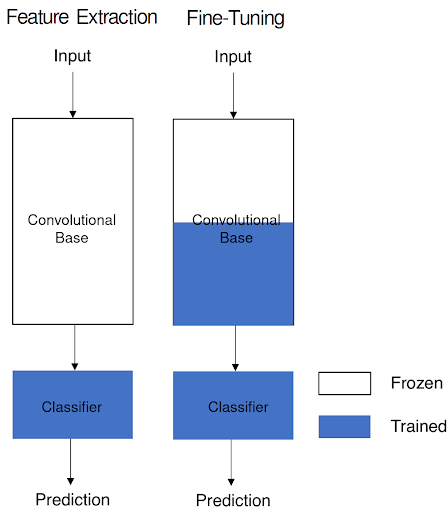Figure 3: Feature Extraction compared to Fine-Tuning.

It is important to point out that, according to F. Chollet, the top layers of a pre-trained convolutional base can be fine-tuned only if the classifier on top of it has already been previously trained. The reason is that if the classifier was not already trained, then its weights would be randomly initialized. As a consequence, the error signal propagating through the network during training would be too large and the unfrozen weights would be updated disrupting the abstract representations previously learned by the convolutional base.

#deep-learning #machine-learning #artificial-intelligence #image-classification #transfer-learning #deep learning1596178620

## Learn Transfer Learning for Deep Learning by implementing the project.

Project walkthrough on Convolution neural networks using transfer learning

From 2 years of my master’s degree, I found that the best way to learn concepts is by doing the projects. Let’s start implementing or in other words learning.

## Problem Statement

Take an image as input and return a corresponding dog breed from 133 dog breed categories. If a dog is detected in the image, it will provide an estimate of the dog’s breed. If a human is detected, it will give an estimate of the dog breed that is most resembling the human face. If there’s no human or dog present in the image, we simply print an error.

Let’s break this problem into steps

1. Detect Humans
2. Detect Dogs
3. Classify Dog breeds

For all these steps, we use pre-trained models.

Pre-trained models are saved models that were trained on a huge image-classification task such as Imagenet. If these datasets are huge and generalized enough, the saved weights can be used for multiple image detection task to get a high accuracy quickly.

## Detect Humans

For detecting humans, OpenCV provides many pre-trained face detectors. We use OpenCV’s implementation of Haar feature-based cascade classifiers to detect human faces in images.

``````### returns "True" if face is detected in image stored at img_path
def face_detector(img_path):
gray = cv2.cvtColor(img, cv2.COLOR_BGR2GRAY)
return len(faces) > 0
``````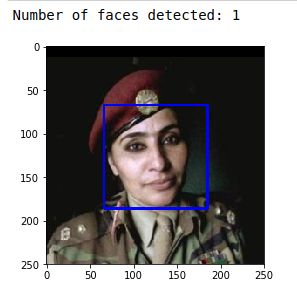## Detect Dogs

For detecting dogs, we use a pre-trained ResNet-50 model to detect dogs in images, along with weights that have been trained on ImageNet, a very large, very popular dataset used for image classification and other vision tasks.

``````from keras.applications.resnet50 import ResNet50

### define ResNet50 model
ResNet50_model_detector = ResNet50(weights='imagenet')
### returns "True" if a dog is detected
def dog_detector(img_path):
prediction = ResNet50_predict_labels(img_path)
return ((prediction <= 268) & (prediction >= 151))
``````

## Classify Dog Breeds

For classifying Dog breeds, we use transfer learning

Transfer learning involves taking a pre-trained neural network and adapting the neural network to a new, different data set.

To illustrate the power of transfer learning. Initially, we will train a simple CNN with the following architecture: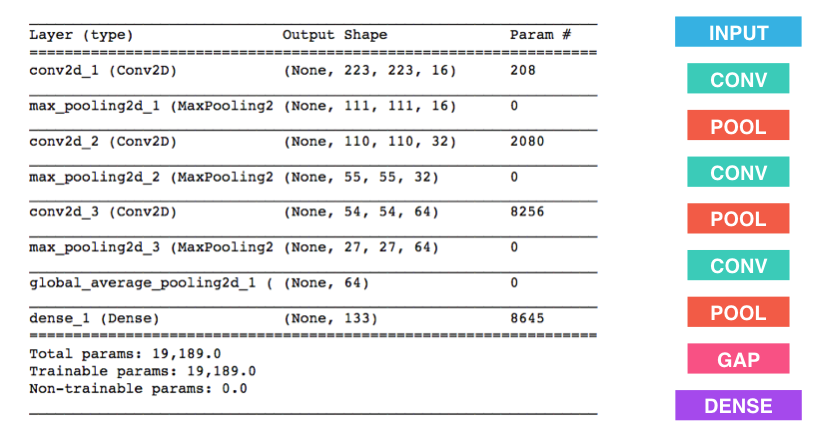Train it for 20 epochs, and it gives a test accuracy of just 3% which is better than a random guess from 133 categories. But with more epochs, we can increase accuracy, but it takes up a lot of training time.

To reduce training time without sacrificing accuracy, we will train the CNN model using transfer learning.

#data-science #transfer-learning #project-based-learning #cnn #deep-learning #deep learning